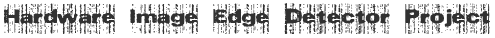Edge Detection in FPGA using Sobel Operator

### Sobel Core Module Verilog Code

The following is the verilog code for the core sobel module in our project.

```/* Verilog code to calculate Sobel
* Free to download modify and use
*
*/
module sobel( p0, p1, p2, p3, p5, p6, p7, p8, out);

input  [7:0] p0,p1,p2,p3,p5,p6,p7,p8;	// 8 bit pixels inputs
output [7:0] out;					// 8 bit output pixel

wire signed [10:0] gx,gy;    //11 bits because max value of gx and gy is
//255*4 and last bit for sign
wire signed [10:0] abs_gx,abs_gy;	//it is used to find the absolute value of gx and gy
wire [10:0] sum;			//the max value is 255*8. here no sign bit needed.

assign gx=((p2-p0)+((p5-p3)<<1)+(p8-p6));//sobel mask for gradient in horiz. direction
assign gy=((p0-p6)+((p1-p7)<<1)+(p2-p8));//sobel mask for gradient in vertical direction

assign abs_gx = (gx? ~gx+1 : gx);	// to find the absolute value of gx.
assign abs_gy = (gy? ~gy+1 : gy);	// to find the absolute value of gy.

assign sum = (abs_gx+abs_gy);				// finding the sum
assign out = (|sum[10:8])?8'hff : sum[7:0];	// to limit the max value to 255

endmodule```
Download | Licensed under GPL Public License | In short free code

Tags: Verilog , Sobel ,